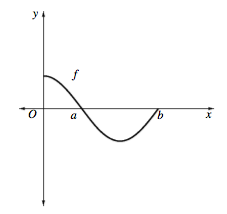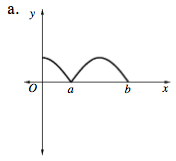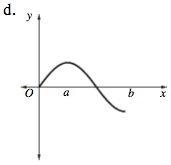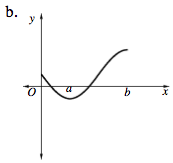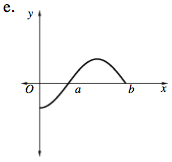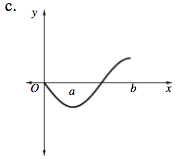### Home > CALC > Chapter 8 > Lesson 8.3.1 > Problem8-107

8-107.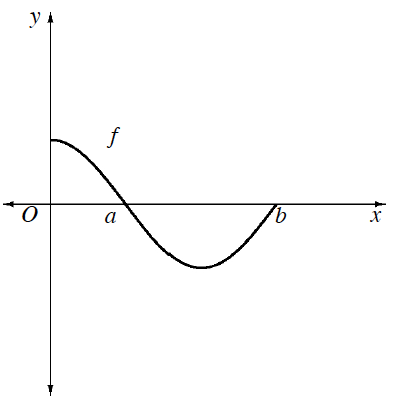Multiple Choice: Given the graph of f at right and g(x) =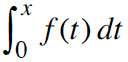. Which of the graphs below could be the graph of g? Homework Help ✎

 a.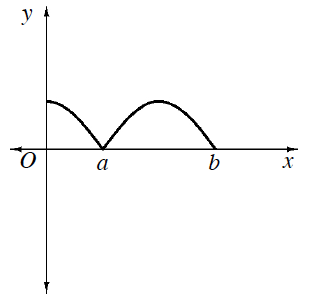b.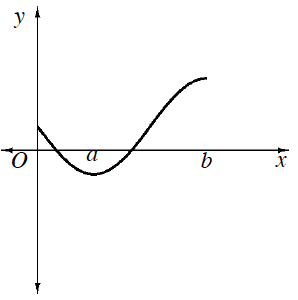c.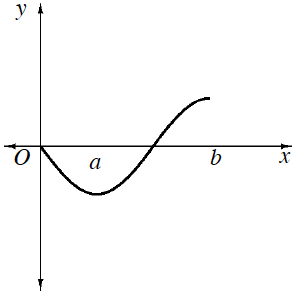d.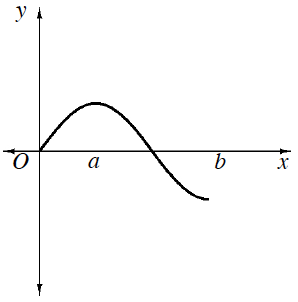e.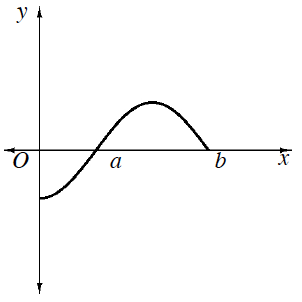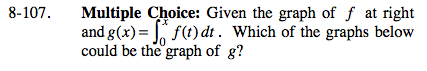g(x) represents the accumulated area under y = f(t). Is that accumulated area ever negative? If so, where?